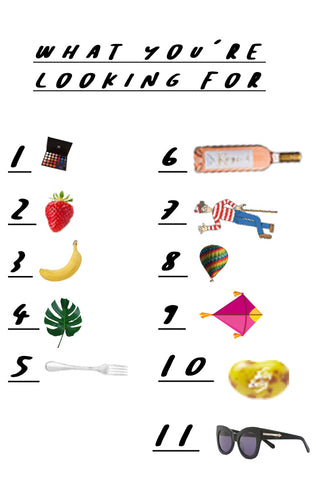# MY SPY

Find the items below in the grid. Note their location in the grid using the letters and numbers eg. 6b. Each letter is worth a certain amount (see below). Add the numbers value to the other coordinate. For example. 6B would be b=22 so 22+6 = 28 Therefore the digits to add to your code would be 28. The code goes in the order of images below. Once you have cracked the code, enter it into the discount code section - if you are successful it will take 100% off. If it has been take the item will say 'SOLD OUT'.

A=47 B=22 C=38 D=23 E-58 F=13 G=9 H=77 I=41 J=82 K=2 L=44 M=9 N=21 O=12 P=43